MS Excel has a practical are or application in almost every field. Be it Engineering, Banking, Accounting, Medicine, etc. It is good you know the practical, real life or business applications of Microsoft Excel because it will help you to know the area of MS Excel that relates to your own field and then concentrate more in that area. You should also master some basic Excel operators and their order of precedence, built-in functions, function keys, Error Messages and their solutions, etc.

This is the third part of this chapter. It is recommended that you first read part one and two before reading this part. If you have not read part read part one, Click to read Part 1: Introduction to MS-Excel.

## TUTORIAL CONTENTS

• OPERATORS
• TYPES OF OPERATORS
• ENTERING A FORMULA
• ORDER OF PRECEDENCE
• TYPES OF FORMULA IN RELATION TO CELL REFERENCING
• FUNCTION AND MS-EXCEL BUILT-IN WORKSHEET FORMULAS OR FUNCTIONS
• EXCEL FUNCTION KEYS
• MS-EXCEL ERROR MESSAGES, CAUSES, SOLUTIONS AND SOME ERROR DEBUGGING FUNCTIONS
• BUSINESS APPLICATIONS OF SOME MS-EXCEL ARITHMETIC OR MATHEMATICAL FUNCTIONS
• EXERCISE 1
You can download the PDF e-book file of this chapter for free and study at your convenient time. The e-book contains both parts of this chapter properly written. Click to download the e-book: Fundamentals of MS-Excel Using MS-Excel – 2016, 2013,2010 and 2007.

## OPERATORS

These are signs or symbols used in MS-Excel to perform calculations and to define criteria or conditions in some functions.

### TYPES OF OPERATORS

There are three main types of operators. They include:

•    Mathematical or arithmetic operators
•    Relational or Comparison operators
•    Logical or Conditional operators
•    Text operators
•    Reference operators

#### Mathematical or Arithmetic Operators

These are the operators used to perform calculations. They include:

•    Addition sign (+) e.g. the formula, =10+5 outputs 15
•    Subtraction sign (-) e.g. the formula, =10-5 outputs 5
•    Multiplication sign (*) e.g. the formula, =10*5 outputs 50
•    Division sign (/) e.g. the formula, =10/5 outputs 2
•    Exponential sign (^) e.g. the formula, =10^5 outputs 100000
•    Negation sign (-) e.g. the formula, =-10 outputs –10
•    Percentage sign (%) e.g. the formula, =10% outputs 0.1

#### Relational or Comparison Operators

These are the operators used to define criteria or conditions in some functions or formulas .They include:

•    Equality sign also referred to as Equal to (=) e.g. the formula, =10=5 returns FALSE
•    Inequality sign also referred to as Not Equal to (<>) e.g. the formula, =10<>5 returns TRUE
•    Greater than (>) e.g. the formula, =5>10 returns FALSE
•    Less than (<) e.g. the formula, =10<5 returns FALSE
•    Greater than or Equal to (>=) e.g. the formula, =5>=10 returns FALSE
•    Less than or Equal to (<=) e.g. the formula, =5<=10 returns TRUE

#### Logical or Conditional Operators

They are used together with some text and relational operators to define conditions in some functions. They are mainly used in logical functions e.g. IF function.
Some commonly used ones include:

•    AND
•    OR
•    NOT
•    NOR
•    NONE

#### Text Operators

They are used to manipulate text or label cells. Example of a text operator is the ampersand (&) operator which is used to concatenate text strings. For example, if you enter the formula ="john"&"son" into a cell, Excel displays software.

Note that the quotation marks and the ampersand aren’t shown in the result. You also can use ampersand to combine cells that contain text. For example, if A1 contains the text Mary and A2 contains Johnson, entering the formula =A1&" and "&A2 returns Mary and Johnson. We will apply this operator in chapter five under Referencing Data and Formulas in Other Worksheets of a Workbook.

#### Reference Operators

The reference operators combine two cell references or ranges to create a single joint reference. They include:

•    Range (colon): Produces a range from two cell references for example, A1:C5.

•    Intersection (space): Produces a range that is the intersection of two ranges for example, A1:C5 B2:E8.

•    Union (comma): Produces a range that is the union of two ranges for example, A1:C5,B2:E8.

## ENTERING A FORMULA IN EXCEL WORKSHEET

A formula is an expression used to calculate a value. You can enter a formula by typing the expression into the required cell. If the value in a column is calculated using the same formula, you can make use of auto fill or fill handle to reduce the stress of typing in individual cells of the column. You can also enter a formula inside the formula bar by first activating the output cell

Form MS-Excel to treat an expression as a formula, it must begin with an equality sign (=), else, Excel treats it as a text or label. An expression can contain more than one arithmetic operator that applies to either values or cell addresses in a workbook. For example, assuming cell A2 has the value 3 and cell B3 has a value 4, if you wish to perform calculation using these values in another cell, you can type =3*4 or =A2*B3 (This is cell referencing, I will elaborate more on it shortly) in the output cell.

## ORDER OF PRECEDENCE

This explains the hierarchy of operators, especially mathematical or arithmetic operators in a formula. It is a set of pre-defined rules that MS-Excel follows to unambiguously evaluate a formula by determining the operator to apply first, second, etc. especially in a complex formula. Below is a list of operators and their order of preference in Excel.

•    Range (:) - 1st
•    Intersection (<space>) - 2nd
•    Union (,) - 3rd
•    Negation (-) - 4th
•    Percentage (%) - 5th
•    Exponentiation (^) - 6th
•    Multiplication and Division (* and /) - 7th
•    Addition and Subtraction (+ and -) - 8th
•    Concatenation (&) - 9th
•    Comparison operators (=, <, >, <=, >=, <>) - 10th

#### NOTE:

If an expression contains two or more operators with the same level of precedence or hierarchy, Excel applies or evaluates them going from left to right in the expression. You can add parenthesis to change the order of evaluation since parenthesis has the highest precedence, Excel execute any operator enclosed in a parenthesis first.

### ILLUSTRATION FOR ORDER OF PRECEDENCE

1.    The expression, 5+3*2 outputs the value, 11 as the result. Because multiplication has precedence over addition, Excel first evaluates 3*2 and then adds 5.

2.    The expression, 4*3/2 outputs the value, 6 as the result. The two operators have the same level of precedence, so Excel evaluates the expression going from left to right i.e. multiplication before addition.

3.    The expression (5+3)*2 outputs the value, 16 as the result. Although multiplication has precedence over addition, addition was evaluated first because it is enclosed in parenthesis, which has the highest precedence.

## TYPES OF FORMULA IN RELATION TO CELL REFERENCING IN MS EXCEL

Categorizing formulas with respect to cell referencing, there are three types of formula. They include:

### RELATIVE CELL REFERENCE FORMULA

This is the type of formula in which the cell references or addresses in it change with respect to new location. For example, if you copy a formula that contains a relative cell address, A5 to a new location, say three rows down and five columns to the right, the relative cell address, A5 in the formula changes to F8. It is activated by default.

### ABSOLUTE CELL REFERENCE FORMULA

In this type of formula, the cell references or addresses in the formula does not change with respect to a new location. To create an absolute cell reference in a formula, you prefix the column and row designation with a dollar sign (\$).This tells Excel that you want to use an absolute reference.

When you refer to a cell in a formula using the absolute reference format, Excel uses the physical address of the cell. For example, Excel interprets the formula =\$A\$1*2 as “Multiply the contents of cell A1 by 2.” No matter where you copy or move this formula, the cell reference doesn’t change. The cell address is said to be anchored.

### MIXED CELL REFERENCE FORMULA

In this type of formula, the precedent cell (another cell address in a formula) has the characteristics of both relative and absolute cell reference.
For example, a mixed cell reference for cell A5 would either be written as \$A5 or A\$5. In the case of \$A5, the row reference would shift, but the column reference would not shift when the formula is copied to a new location. In the case of A\$5, only the column reference will shift if the formula is copied to a new location.

#### NOTE:

You can switch between absolute relative or mixed cell reference in a formula by selecting the formula and then pressing the F4 key on your keyboard repeatedly.

## FUNCTION AND MS-EXCEL BUILT-IN WORKSHEET FORMULAS OR FUNCTIONS

A function is a named block of code that performs a specific task, possibly acting upon a set of values given to it, or parameters, and possibly returning a single value. It is a sequence of values, cell references, names, functions, or operators in a cell that together produce a new value. A formula always begins with an equality sign (=).

Regardless of their source, all functions are evaluated in the same way. In MS-excel and some programming languages, a function takes the format: =function_name ([parameter [,...] ] ).
Excel built-in worksheet functions are specialized formulas or functions that are embedded in MS-Excel which enables the user to perform calculations easily and automatically.

### PARTS OF BUILT IN FUNCTIONS

There are three parts of functions in MS-Excel. They include:

#### 1.    EQUALITY SIGN (=):

It tells Excel that you want to perform a calculation.

#### 2.    FUNCTION PARAMETER OR ARGUMENT:

This specifies the range of data you want to calculate or the values the function requires to evaluate correctly. The number of parameters a function requires differs from function to function (and, as we’ll see later, may even vary for the same function).

The parameters or argument supplied to the function may be any valid expression and should be in the specific order expected by the function. They are values that provides information to an action, an event, a method, a property, a function, or a procedure.

A function’s documentation will tell you what parameters the function expects and what values you can expect to be returned. It must be enclosed in parenthesis.

#### 3.    FUNCTION NAME:

When you type an equality sign followed by the function name of a built-in function in Excel, it will brief you about the function and the type of parameters needed by a function.

### CATEGORIES OF MS-EXCEL BUILT-IN WORKSHEET FORMULAS / FUNCTIONS

MS-Excel built-in functions have about thirteen (13) main categories. They include:

•    Compatibility functions
•    Cube functions
•    Database functions
•    Date and time functions
•    Engineering functions
•    Financial functions
•    Information functions
•    Logical functions
•    Lookup and reference functions
•    Math and trigonometry functions
•    Statistical functions
•    Text functions
•    User defined functions that are installed with add-ins

### HOW TO SEARCH FOR MS-EXCEL FUNCTIONS AVAILABLE TO YOUR OWN VERSION

The function types under each category vary with respect to the version of MS –Excel you are using.

#### To view a list of the functions available in your own version of Excel:

1.    Open MS-Excel. Locate and click the Excel Help icon (?). It is normally located the window control box.Excel help icon
2.    This opens the MS-Excel Help window. Type Excel function by category in the search bar and press the enter key.

3.    From the search result, click the Excel functions (by category) link as shown below.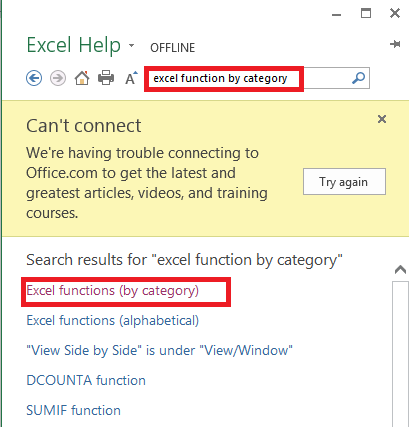MS-Excel function search results
4.    Excel Help displays links to the worksheet function categories. Click any of the links to see all the functions under that category. You can also click any function to learn more about it.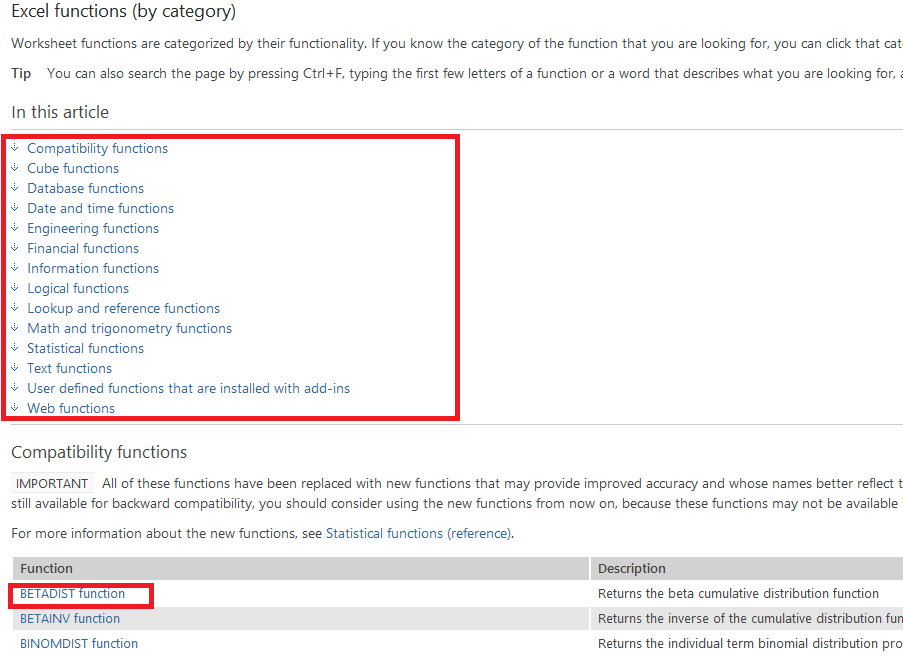MS-excel function category links

## EXCEL FUNCTION KEYS

These are the special keys used in MS-Excel to issue a command or order without going through the normal procedure i.e. they provide shortcuts and save time.
These keys range from F1 to F12. They include:

#### F1 (HELP AND SUPPORT)

This key helps you to get help from windows or internet about any command or topic of your choice.

#### F2 (EDIT)

This key is used to edit the contents of an active cell.

#### F3 (NAME)

This key is used to create or display created range of names so as to paste any of them into a formula.

#### F4 (ABSOLUTE)

This key is used to create an absolute cell in a formula.

#### F5 (GO TO)

This key is used to move the cell pointer to the specified address.

#### F6 (WINDOW)

This key is used to move the cell pointer from one worksheet window to another any time the worksheet window is split into two or more windows.

#### F7 (SPELLING)

This key is used to spell check a worksheet content.

#### F8 (SELECTION)

This key is used to extend selection.

#### F9 (CALCULATION)

This key is used to calculate all the worksheet opened in a workbook.

This key is used to activate the menu bar.

#### F11 (CHART)

This key is used to create an automatic chart in the selected or activated worksheet.

#### F12 (SAVE AS)

This key is used to save a new workbook for the first time or save an existing worksheet as a new workbook.

Here is a Free MS Excel Shortcut Keys and Formulas App

## MS-EXCEL ERROR MESSAGES, CAUSES, SOLUTIONS AND SOME ERROR DEBUGGING FUNCTIONS

These are error messages or alerts that displays when it discovers an error in a formula or cell address. They include the following:

### #REF!

Excel displays this error message when a cell address or reference is not valid i.e. deleting cells referred to by other formulas.

#### Solution:

Click undo from the quick access tool bar of press the Ctrl + Z keys.

### #NAME?

Excel displays this error message when the name of a function is mis spelt.

#### Solution:

Correct the spelling.

### DIV/0!

Excel displays this error message when it tries to divide by zero or if a referenced cell is blank when it is required to have a value.

#### Solution:

Fix the formula if it is referring to the wrong cell or fix data in the reference cell.

### #N/A

This error message means Not Available. Excel inserts this error value in the active cell when you have improperly used some certain function argument.

#### Solution:

Check the formula of function argument you are using.

### ######

Excel inserts this error value in the current cell that contains a value wider than the sell width.

#### Solution:

Adjust or increase the column width.

### #NUM!

Excel displays this error message when you try to use an unacceptable numeric argument such as calculating the square root of a negative number.

### #VALUE!

This error message is displayed when the wrong type of argument or operand is used. For example, entering a text when the formula requires a number or logical value or operator.

#### Solution:

Ensure that the formula contains the correct argument.

### #NULL!

This error message is displayed when you join two areas that should be separated.

#### Solution:

Use a comma sign (,) to separate the areas.

### SOME ERROR DEBUGGING FUNCTIONS

These are some Excel built-in functions that are used to handle errors. They are also used to instruct MS-Excel how, when and which error message to display. Some commonly used ones are:

#### •    ERROR.TYPE function

This function returns a number corresponding to one of the error values in Microsoft Excel or returns the #N/A error if no error exists. You can use ERROR.TYPE in an IF function to test for an error value and return a text string, such as a message, instead of the error value.
Syntax: =ERROR.TYPE(error_val)

The ERROR.TYPE function syntax has the following arguments:
Error_val (required): This is the error value whose identifying number you want to find. Although error_val can be the actual error value, it will usually be a reference to a cell containing a formula that you want to test.
If error_val is #NULL! ERROR.TYPE returns 1
If error_val is #DIV/0! ERROR.TYPE returns 2
If error_val is #VALUE! ERROR.TYPE returns 3
If error_val is #REF! ERROR.TYPE returns 4
If error_val is #NAME? ERROR.TYPE returns 5
If error_val is #NUM! ERROR.TYPE returns 6
If error_val is #N/A ERROR.TYPE returns 7
If error_val is #GETTING_DATA  ERROR.TYPE returns 8
If error_val is anything else ERROR.TYPE returns #N/A

#### •    IFERROR function

This function returns a value you specify if a formula evaluates to an error; otherwise, returns the result of the formula. Use the IFERROR function to trap and handle errors in a formula.
Syntax: IFERROR(value, value_if_error)
The IFERROR function syntax has the following arguments (argument: A value that provides information to an action, an event, a method, a property, a function, or a procedure.):

Value    Required: The argument that is checked for an error.

Value_if_error (required). The value to return if the formula evaluates to an error.

The following error types are evaluated: #N/A, #VALUE!, #REF!, #DIV/0!, #NUM!, #NAME?, or #NULL!.

#### REMARKS:

If Value or Value_if_error is an empty cell, IFERROR treats it as an empty string value ("").
If Value is an array formula, IFERROR returns an array of results for each cell in the range specified in value.

#### NOTE:

For a clearer, detailed explanation of these error messages and error debugging built-in functions with illustration, click the help icon, located top right near the window control box. Type the name of the error message in the search bar e.g. #REF or IFERROR function and press the enter key. Click the error message article link to see full details.

## BUSINESS APPLICATIONS OF SOME MS-EXCEL ARITHMETIC OR MATHEMATICAL FUNCTIONS

In this section, I will show you the practical business application of some Excel arithmetic functions.

### ILLUSTRATION 1: SALES REPORTSales Report Question - table1
From the table above, calculate:
1.    Unit profit for each product
2.    Total cost price for each product
3.    Total selling price for each product
4.    Total profit for each product

### SOLUTION

Create the table in Excel. You may wish to place the title SALES REPORT in the first row by first merging the cells in the row. Note that your cell reference and mine will vary because you have moved a row down. i.e. if my formula contains the cell address B2, your will now be B3. You can also specify the data format for each column.

Type the following formulas in the appropriate columns as indicated.
1.    TOTAL COST PRICE=D2-C2 enter key i.e. UNIT S.P. minus UNIT C.P.

#### NOTE:

You can specify the cell references by clicking the appropriate cells. For example, for the formula above,  type the equality sign, then click on cell D2 followed by minus sign, then click on cell C2 and press the enter key. Use the auto fill or fill handle to fill the subsequent cells of the column.

2.    TOTAL COST PRICE=B2*C2 enter key i.e. QTY*UNIT C.P.

3.    TOTAL SELLING PRICE=B2*D2 enter key i.e. QTY*UNIT S.P.

4.    TOTAL PROFIT=B2*E2 enter key i.e. QTY*UNIT PROFIT.

Below is the complete table. Yours should look like this if got everything right.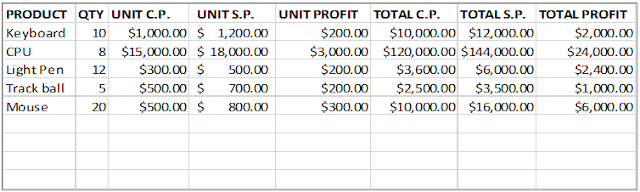Sales Report Solution - table1

Now let us go over to the second practical illustration.

### ILLUSTRATION 2: PERCENTAGE DISCOUNTPercentage Discount Question-table2
From the table above, calculate the:
2.    Actual unit cost price

#### SOLUTION

Create the table in Excel. You may wish to place the title PERCENTAGE DISCOUNT in the first row by first merging the cells in the row. Note that your cell reference and mine will vary because you have moved a row down. i.e. if my formula contains the cell address B2, your will now be B3. You can also specify the data format for each column.

Type the following formulas in the appropriate columns as indicated:

1.    DISCOUNT RECEIVED =C2*5% enter key.

2.    ACTUAL UNIT COST PRICE =C2-D2 enter key.

Below is the complete table. Yours should look like this if got everything right.Percentage Discount Solution-table2
Now let us go over to the second practical illustration.

### ILLUSTRATION 3: PERCENTAGE INCREMENT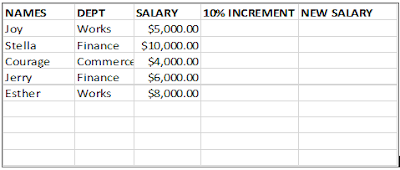Percentage Discount Question-table3
From the table above, calculate the:

1.    10% salary increment
2.    The new salary

### SOLUTION

Create the table in Excel. You may wish to place the title PERCENTAGE INCREMENT in the first row by first merging the cells in the row. Note that your cell reference and mine will vary because you have moved a row down. i.e. if my formula contains the cell address B2, your will now be B3. You can also specify the data format for each column.

Type the following formulas in the appropriate columns as indicated:

1.    DISCOUNT RECEIVED =C2*10% enter key.

2.    ACTUAL UNIT COST PRICE =C2+D2 enter key.

Below is the complete table. Yours should look like this if got everything right.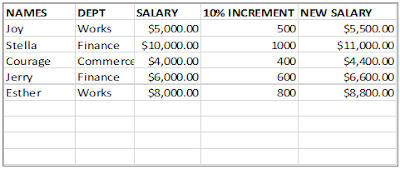Percentage Discount Solution-table3
Below are some exercises for you. Try them out on your own.

## PRACTICAL EXCEL EXERCISE FOR CHAPTER 1

Please endeavour to try these real life questions as it will help to have a deeper knowledge of the contents of this chapter.
1.
a.    What is an electronic spreadsheet?
b.    List any five spreadsheet package you know.

2.    Briefly explain the following:
a.    Workbook
b.    Worksheet
c.    Cell
e.    Cell pointer

3.    Define operators and list the types of operators.

4.    Explain briefly with good illustrations, order of precedence.

5a.    What are MS-Excel built-in formulas?
b.    List the categories of Excel built formulas

6.    Write and explain the built-in functions to perform the following:
a.    2+4+6+10
b.    2*4*6*10
c.    A1+B1+C1/3
d.    C5^2

7.    Explain the functions of the following function keys in MS-Excel
a.    F2
b.    F5
c.    F7
d.    F10
e.    F11

8.    Explain the causes of the following error messages and their solution.
a.    #VALUE!
b.    #REF!
c.    #####
d.    #NUM!

9a.    Prepare a sales report for Mr. Biggs fast food with the following table information below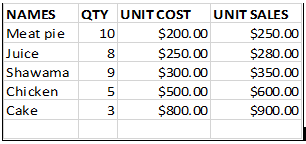Mr biggs sales-report-exercise-table1
b.    As spreadsheet manager, calculate the following:
i.    Unit profit
ii.    Total cost
iii.    Total sales
iv.    Total profit
v.    Calculate the discount of 5% on each sale
vi.    Calculate the actual selling price
vii.    Calculate the new profit
viii.    Calculate the total discount

This is the end of this chapter.

## Recommended Entrepreneurship BooksThe Passionate Entrepreneur’s Strategies: Learn how to discover business ideas that will be successful, grow multiple online income streams, make money from home, attract new and retain customers, applications of SWOT analysis in any business, skills that will help you succeed as an entrepreneur, self-discipline, etc. Lucrative Business Ideas Series Book 1. By Buzzer Joseph. Read more about the book.How to Make Money from Blogging and Affiliate Marketing: Learn the Step by Step Guide of Creating, Growing and Optimizing your WordPress Blog from Scratch for Search Engines (SEO) and How to Make Money through Google AdSense, Affiliate Marketing and Other Strategies for Earning Passive Income Online. Lucrative Business Ideas Series Book 2. By Buzzer Joseph. Read more about the book.How to Make Money Self-Publishing Kindle Books for Beginners: Learn the Step by Step Guide to Self-Publish a Best Selling Book on Amazon KDP, How to Design Book Covers with Adobe Photoshop, Format, Edit and Proofread your Book Manuscript with Microsoft Word, Promote your Book on Various Online Platforms and Earn Monthly Passive Income Online. Lucrative Business Ideas Series Book 3. By Buzzer Joseph. Read more about the book.Complete Solar PV System Installation Guide: DIY guide on how to install grid-connected and off-grid solar PV systems, how to build solar-powered electric cars, convert conventional cars to electric cars, online and offline ways to make money from solar energy sector (including stocks), electrical wiring and protection system installation guides, plus free resources for skill acquisition and personal development. Lucrative Business Ideas Series Book 4. By Buzzer Joseph. Read more about the book.How to Make Money from Crypto Trading and Investing for Beginners: Step by step guide with screenshots on the basics of blockchain technology, bitcoin and altcoins, crypto security tips, cryptocurrency market analysis (FA and TA), long term and short term trading, how to calculate your profits in excel, legit ways to make money with cryptocurrencies, DeFi yield farming and staking, how to use any centralized and decentralized exchange platform and more, plus crypto investment bonus! Lucrative Business Ideas Series Book 5. By Buzzer Joseph. Read more about the book.Blockchain Practical Guide: Step by step guide with pictures, on how to monetize the latest blockchain technology trends: crypto trading and tools, especially Ethereum, DeFi, Staking, NFTs, Top 100 Play-to-Earn (P2E) and NFT games, Web 3.0, Metaverse, Virtual Reality (VR), Augmented Reality (AR), blockchain job opportunities, with crypto investment bonus! Lucrative Business Ideas Series Book 6. By Buzzer Joseph. Read more about the book.How to Make Money with Blockchain Web3 Apps: Step by Step Guide with Screenshots and Videos on How to Make Money from Blockchain and Web3 Projects – NFTs, Play-to-Earn (P2E) Games, Move-to-Earn (M2E) Apps, Watch-to-Earn (W2E) Apps, SocialFi and GameFi Apps, with crypto investment bonus! Lucrative Business Ideas Series Book 7. By Buzzer Joseph and Kelly Joseph. Read more about the book.

1.2.